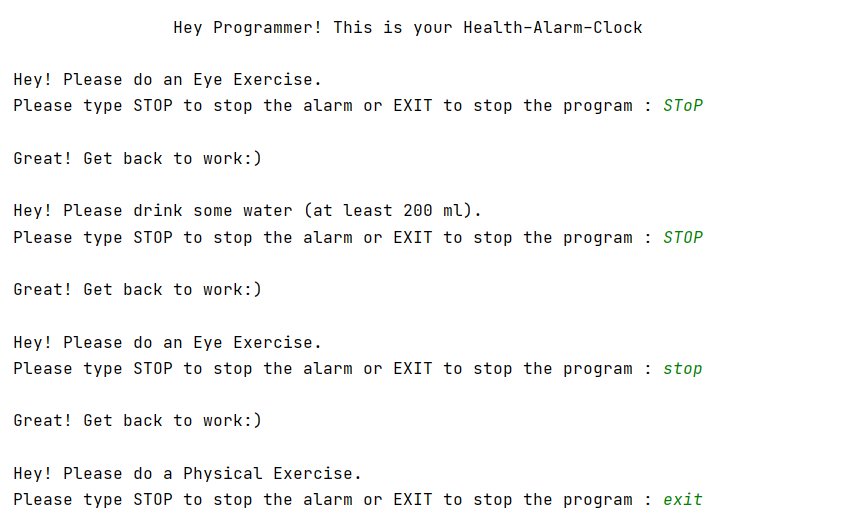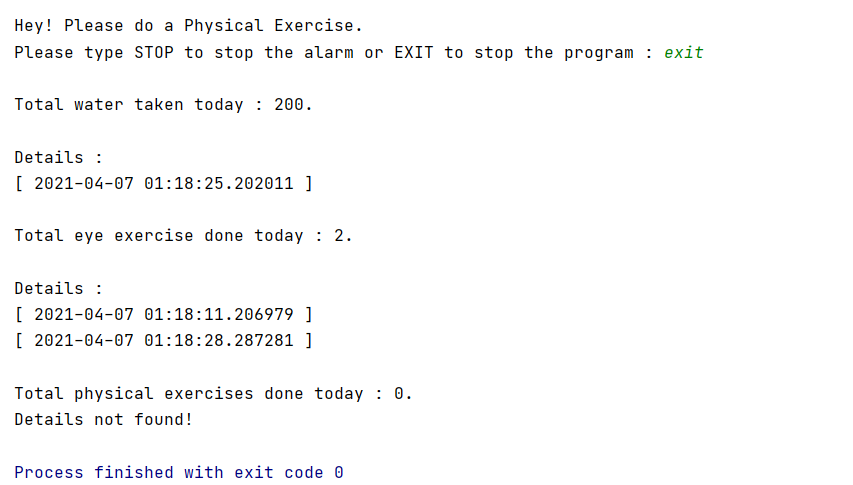Open in App
Not now

# Create Health Clock for programmers using Python

• Difficulty Level : Easy
• Last Updated : 25 Nov, 2022

In this article, we will be creating a Python script that takes care of the health of a person, especially a programmer. It will remind the user to drink water, do an eye exercise, and do a physical exercise at different specified time intervals. It will also keep a log of time at which the exercises are done, water is drunk as well as the no of exercises and quantity of water taken. At the end of the program, the whole stats will be shown along with time stamps.

Approach:

• We will import mixer from pygame module to play our sound, time from time module to set the time interval and sleep from time module to freeze the program for a respective time interval.
• We will define two functions- getdate() that will return the current date and time when the function is called, and musicloop() that will play the given file.
• After that, we will initialize the water, eye exercise, and physical exercise variable with the current time. We are doing this so that we can play the sound at the equal desired time interval.
• Now, we will be running an infinite while loop that will contain three if conditions (one for each i.e. water, eye exercise, and physical exercise).
• In each of the if conditions, we will be doing the same thing i.e. calling the musicloop() function to play music, asking the user to do a respective thing to stop music, and noting the time (of completion of exercise) in a file so that it can be shown at last.
• We will also increment no of exercises and value of water drank so that we can print it at the end of the program.
• At last, we are using file handling to display all the above-mentioned data.

Below is the Implementation.

## Python3

 `# import required modules``from` `pygame ``import` `mixer``from` `time ``import` `time``from` `time ``import` `sleep`  `def` `getdate():` `    ``# To get the current date and time``    ``# at time of entry``    ``import` `datetime``    ``return` `(``str``(datetime.datetime.now()))`  `def` `musicloop(stopper):``    ``mixer.init()``    ``mixer.music.load(``"music.mp3"``)` `    ``# playing the music provided i.e. music.mp3``    ``mixer.music.play()``    ``while` `True``:``        ``x ``=` `input``(``            ``"Please type STOP to stop the alarm or EXIT to stop the program : "``)` `        ``# music termination condition.``        ``if` `(x.upper() ``=``=` `stopper):``            ``print``(``"\nGreat! Get back to work:)\n"``)``            ``mixer.music.stop()``            ``return` `True``            ``break` `        ``# program termination condition.``        ``elif` `(x.upper() ``=``=` `"EXIT"``):``            ``return` `False`  `# variables initialized with 0 for counting total``# number of exercises and water drank in a day``total_water ``=` `0``total_physical_exercises ``=` `0``total_eye_exercises ``=` `0` `if` `__name__ ``=``=` `'__main__'``:``    ``print``(``"\n\t\t\t\tHey Programmer! This is your Health-Alarm-Clock\n"``)``    ``time_phy ``=` `time()``    ``time_drink ``=` `time()``    ``time_eyes ``=` `time()` `    ``eyes_delay ``=` `10``    ``drink_delay ``=` `20``    ``phy_delay ``=` `35` `    ``while``(``True``):` `        ``# Drink water condition.``        ``if` `(time() ``-` `time_drink > drink_delay):``            ``print``(``"Hey! Please drink some water (at least 200 ml)."``)` `            ``# Checking the user input so that music``            ``# can be stopped.``            ``if``(musicloop(``"STOP"``)):``                ``pass``            ``else``:``                ``break` `            ``# reinitializing the variable``            ``time_drink ``=` `time()` `            ``# incrementing the value``            ``total_water ``+``=` `200` `            ``# opening the file and putting the data``            ``# into that file``            ``f ``=` `open``(``"drank.txt"``, ``"at"``)``            ``f.write(``"[ "` `+` `getdate() ``+` `" ] \n"``)``            ``f.close()` `        ``# Eye exercise condition.``        ``if` `(time() ``-` `time_eyes > eyes_delay):` `            ``print``(``"Hey! Please do an Eye Exercise."``)` `            ``if` `(musicloop(``"STOP"``)):``                ``pass``            ``else``:``                ``break` `            ``time_eyes ``=` `time()``            ``total_eye_exercises ``+``=` `1``            ``f ``=` `open``(``"eye.txt"``, ``"at"``)``            ``f.write(``"[ "` `+` `getdate() ``+` `" ] \n"``)``            ``f.close()` `        ``# Eye exercise condition.``        ``if` `(time() ``-` `time_phy > phy_delay):``            ``print``(``"Hey! Please do a Physical Exercise."``)` `            ``if` `(musicloop(``"STOP"``)):``                ``pass``            ``else``:``                ``break` `            ``time_phy ``=` `time()``            ``total_physical_exercises ``+``=` `1``            ``f ``=` `open``(``"phy_exer.txt"``, ``"at"``)``            ``f.write(``"[ "` `+` `getdate() ``+` `" ] \n"``)``            ``f.close()` `    ``print``()``    ``print``(f``"Total water taken today : {total_water}."``)` `    ``try``:``        ``f ``=` `open``(``"drank.txt"``, ``"rt"``)``        ``print``(``"\nDetails :"``)``        ``print``(f.read())``        ``f.close()``    ``except``:``        ``print``(``"Details not found!"``)` `    ``print``(f``"Total eye exercise done today : {total_eye_exercises}."``)` `    ``try``:``        ``f ``=` `open``(``"eye.txt"``, ``"rt"``)``        ``print``(``"\nDetails :"``)``        ``print``(f.read())``        ``f.close()``    ``except``:``        ``print``(``"Details not found!"``)` `    ``print``(f``"Total physical exercises done today : {total_physical_exercises}."``)` `    ``try``:``        ``f ``=` `open``(``"phy_exer.txt"``, ``"rt"``)``        ``print``(``"\nDetails :"``)``        ``print``(f.read())``        ``f.close()``    ``except``:``        ``print``(``"Details not found!"``)` `    ``sleep(``5``)`

Output:Video Demonstration:

Code Explanation:

1. The code starts by importing the required modules.
2. Next, a function called getdate is defined that will return the current date and time at time of entry.
3. The musicloop function starts by initializing the mixer object with an empty list of songs to play.
4. Then it loads a song from “music.mp3” into its music object using the load method on mixer.music.
5. Finally, in order to stop playing when the user presses a button, another function called stopper is created which takes one argument: whether or not to stop playing music looping after 30 seconds (stopper=True).
6. The code starts by importing all necessary modules for this program before defining a function called getdate which returns today’s date and time as string value at time of entry.
7. MusicLoop() creates an instance of Mixer class with no songs loaded into it then uses its load method to load “music.mp3” file into its own music object using Python’s import statement syntax (from pygame import * ).
8. After loading “music.mp3”, MusicLoop() calls AnalyzeMusic() which loops through each frame in the song and checks if any notes are being played so that they can be stopped when needed (when stopper==True).
9. The code is a function that loops through the song “music.mp3” and plays it continuously.
10. The code imports the required modules for this program to work properly, including pygame and mixer.
11. The code starts by importing the music library.
12. The code then creates a variable called x which is set to input(“Please type STOP to stop the alarm or EXIT to stop the program : “) .
13. This will prompt for an input from the user and check if it’s either stopper or exit.
14. If it is, then we print out “Great!
15. Get back to work:)” and call mixer.music.stop() .
16. Otherwise, we return True .
17. If you enter in anything other than stopper or exit, then we break out of our loop because that means that you want us to end this program (i.e., you’re done with your alarm).
18. The code is a program that plays music.
19. The code starts by playing the music provided i.e. music.mp3 mixer.music.play() while True: x = input( “Please type STOP to stop the alarm or EXIT to stop the program : “) # music termination condition.
if (x.upper() == stopper): print(“\nGreat! Get back to work:)\n”) mixer.music.stop() return True break # program termination condition. elif (x.upper() == “EXIT”): return False
20. The code starts by printing a message to the programmer.
21. The next line is time_phy which is set to the current time in seconds.
22. Next, we have time_drink which is set to the amount of water that was drank during that day and then finally, we have time_eyes which is set to how many times an eye exercise was done during that day.
23. The first if statement checks whether or not __name__ == ‘__main__’.
24. If it does, then it prints out a message saying “Hey Programmer!
26. Then it sets up some variables for counting total physical exercises and total eye exercises as well as drinking water and doing eye exercises respectively.
27. After this, there are two more if statements checking whether or not the user has completed their daily tasks before continuing on with other code.
28. The code is a snippet of the code that will be executed when the program is run.
29. The first line prints out “Hey Programmer!
30. This is your Health-Alarm-Clock” to the screen.
31. The second line sets up three variables: time_phy, time_drink, and time_eyes.
32. These are all initialized with 0 for counting total number of physical exercises and water drank in a day.
33. The code starts by declaring the variables that will be used in the program.
34. The variable time_drink is set to 0, and it will be incremented every second.
35. The variable drink_delay is set to 20 seconds, which means that the user has 20 seconds before they need to drink some water (at least 200 ml).
36. The code then checks if musicloop(“STOP”) was entered into a text box on screen.
37. If so, then the program will stop playing music and pass .
38. Otherwise, it will break out of its loop and reinitialize itself with a new value for time_drink , which is equal to time() .
39. This way, when you enter “STOP” into a text box on screen again later in your program, it won’t just start playing music again without stopping first.
40. After this initial setup of variables happens at line 10-11 of our code snippet above, we have an infinite while loop that starts at line 12-13: while(True): # Drink water condition.
if (time() – time_drink > drink_delay): # Checking the user input so that music can be stopped.
if(musicloop(“STOP”))
The code will continuously loop for 10 seconds and then stop.
41. The code above will also check to see if the user has inputted “STOP” in order to stop the music.
42. If they have, it will break out of the loop and reinitialize the variable time_drink to be set back to 0.
43. The code opens a text file called drank.txt and writes “[ ” + getdate() + ” ] \n” to the file.
44. It then closes the file after it is done writing in it.
45. The code opens an eye exercise text file called eye.txt and writes “[ ” + getdate() + ” ] \n” to the file, then closes that same text file when it is done writing in it as well.
46. Finally, the code opens a physical exercise text file called phy_exer.txt and writes “[ ” + getdate() + ” ] \n” to that same textfile before closing that one as well when finished with its work of writing into it too!
47. The first condition checks if time has passed since opening eyes for more than five minutes (time_eyes) or more than ten minutes (time_phy).
48. If so, then print out a message telling people they should do an Eye Exercise by doing some type of activity such as blinking their eyes rapidly or looking at something bright like a light bulb for thirty seconds straight without taking any breaks from this activity until they feel tired enough to stop doing this activity on their own accord (musicloop(“STOP”)).
49. If not, then break.
50. The code is used to track the number of eye exercises and physical exercises a person has completed.
51. The code uses two files for storing data, “drank.txt” and “eye.txt”.
52. The first file tracks the date and time when an eye exercise was completed and the second file tracks the date and time when a physical exercise was completed.

My Personal Notes arrow_drop_up